# Second variation

Jump to: navigation, search

A special case of the $n$-th variation of a functional (see also Gâteaux variation), generalizing the concept of the second derivative of a function of several variables. It is used in the calculus of variations. According to the general definition, the second variation at a point $x_0$ of a functional $f(x)$, defined on a normed space $X$, is

$$\delta^2 f (x_0, h) = \frac{d^2}{d t^2} f (x_0 + th) |_{t = 0}$$

If the first variation is zero, the non-negativity of the second variation is a necessary, and the strict positivity

$$\delta^2 f (x_0, h) \geqslant \alpha \| h \|^2, \hspace{1em} \alpha > 0$$

a sufficient, condition (under certain assumptions) for a local minimum of $f(x)$ at the point $x_0$.

In the simplest (vector) problem of the classical calculus of variations, the second variation of the functional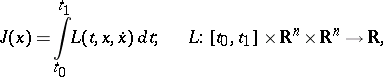considered on the vector functions of classwith fixed boundary values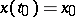,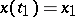, has the form(*)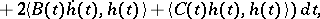where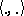denotes the standard inner product in, while,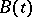,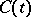are matrices with respective coefficients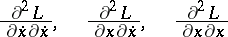(the derivatives are evaluated at the points of the curve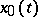). It is expedient to consider the functional ofdefined by (*) not only on the space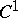, but also on the wider space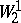of absolutely-continuous vector functions with a square-integrable modulus of the derivative. In this case the non-negativity and strict positivity of the second variation are formulated in terms of the non-negativity and strict positivity of the matrix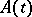(Legendre condition) and the absence of conjugate points (Jacobi condition), which are necessary conditions for a weak minimum in the calculus of variations.

A study of the second variation for extremals which may or may not supply a minimum (but, as before, satisfy the Legendre condition) has been carried out in variational calculus in the large . The most important result was the coincidence of the Morse index of the second variation with the number of points conjugate toon the interval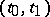.